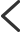游戏分类
• 选择游戏
• 大区
• 服务器

• 穿越火线
• 绝地求生
• 英雄联盟
• QQ飞车
• 逆战
• 穿越火线:枪战王者
• 王者荣耀
• 反恐精英OL
• 地下城与勇士
• 侠盗猎车手Online
• 生死狙击
• 海岛奇兵
• 火线精英
• QQ飞车(手游)
• 和平精英（原刺激战场）
• 武侠乂
A
• Apex英雄
B
• 堡垒之夜
• 部落冲突
C
• 穿越火线
• 穿越火线:枪战王者
• CSGO
• 穿越火线:枪战王者（体验服）
• 彩虹6号
D
• 地下城与勇士
F
• 反恐行动
• 反恐精英OL
G
• 怪物猎人世界
H
• 和平精英（原刺激战场）
• 火线精英
• H1Z1
• 火影忍者（手游）
• 海岛奇兵
J
• 绝地求生
• 纪元1800
• 剑灵
• 僵尸世界大战
• 九阴真经
L
• 口袋版狼人杀
• 黎明杀机
M
• 梦三国
N
• 逆战
• Nba2Kol2
• NBA2KOL
P
• 跑跑卡丁车
• 跑跑卡丁车（手游）
Q
• 全境封锁2
• QQ炫舞手游
• QQ飞车
• 枪神纪
• 全民枪战
• 拳皇命运
• 球球大作战
• QQ炫舞
• QQ飞车(手游)
• QQ游戏
R
• 人类一败涂地
S
• Steam游戏
• 使命召唤OL
• 使命召唤15
• 三国杀手游
• 生死狙击
• 时空猎人
• 生死狙击（手游）
T
• 天涯明月刀
• 天天酷跑
• 坦克世界
W
• 王者荣耀（体验服）
• 武侠乂
• 问道
• 王者荣耀
X
• 侠盗猎车手Online
• 新天龙八部
Y
• 英雄联盟
• 英魂之刃
Z
• 战地风云5
• 战舰世界
• 战地之王
• 只狼：影逝二度